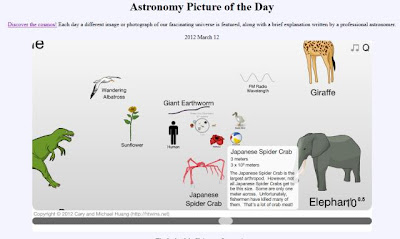## Thursday, 22 March 2012

### Range of measurement (Class 11 - Physics - Ch2)

Click on the figure to go flash animation to see the range of order of length from 10-35 m (Planck length) to 1026m, size of visible universe.courtesy nasa (http://apod.nasa.gov/apod/ap120312.html)
Q1: What is 0.000047 in scientific notation?

Q2: Q: Students X, Y and Z were asked by their teacher to measure the volume of water in a beaker.
X wrote : 200; Y wrote : 200 mL and Z wrote : 200 Lm
Which one of these answers is correct?

Answer: The first one has no units. Therefore, we do not know what does it mean.
The third is also not correct because there is no unit like Lm.
The second one is the only correct answer because it denotes millilitre.

Q3: Convert 10 inches into meters.
: We know that 1 inch = 2.54 cm (centimeters)

Q4: The micrometer (1 μm) is often called the micron.
(a) How many microns make up 1.0 km?
(b) How many microns are in 1.0 yd? (Given 1yd = 0.9144m)
Also mention significant figures while writing the final answers.

(a) Since 1km = 103 m and 1 m = 106 μm
Given 1.0 km has two significant figures,
∴  1.0 km = 103m = (103 m)(106μm) = 109 μm = 1.0 x 109 μm (maintaining two significant figures)

(b) 1.0 yd = (0.91m)(106μm) = 9.1 × 105 μm

Q5: Define parsec unit. Compute  1 parsec into meters (Given, 1 AU = 1.496 x 1011m).
Answer: 1 Parsec (parallactic second) is the distance at which one astronomical unit subtends an angle of 1 second of arc i.e. distance corresponding to annual parallax of 1 sec of arc.courtsey:http://mydarksky.org/2008/04/15/common-stargazing-terms-1/
According to parallax equation, r = l/θ
where l = 1 AU = 1.496 x 1011m and
θ = 1'' = (π radians)(60 x 60 x 180)
r = 1 parsec = 1.496 x 1011 x 60 x 60 x 180/π = 3.08 x 1016m

Q6(cbse paper) : A laser signal is beamed towards a distant planet from the Earth and its reflection is received after seven minutes. If the distance between the planet and the Earth is 6.3 × 1010m, calculate the velocity of the signal.

Answer: Given, Distance (d) = 6.3 × 1010 m
duration time (t) = 7 min = 7 x 60 = 420 secs
If d is the distance of the planet, then total distance traveled
by the signal is 2d.
∴ velocity = (2d)/t = (2 x 6.3 × 1010 m)/ 420 = 3 x 108 m/s

Q7: If the mass of the sun is 2 × 1030 kg and the mass of a proton is 2 × 10-27 kg. If the sun was
made only of protons, calculate the number of protons in the sun?

Given,
Mass of the sun = 2 × 1030 kg
Mass of a proton = 2 × 10-27 kg
∴  Number of protons in the sun = (2 × 1030 ) ÷ (2 × 10-27 kg) = 1057

Q8: Young’s modulus of a metal is 19 x 1010 N/m2 . Express it in dyne/cm2.

Given Young's modulus E =  19 x 1010 N/m= 19 x 1010 kg m s-2 x m-2    (1 N = 1kg m/s2 )
E =  19 x 1010 kg m-1 s-2 =  19 x 1010 x 103g x (102cm)-1 s-2
E = 19 x 1010+3-2 g cm-1 s-2 = 19 x 1011 g cm-1 s-2
E = 19 x 1011 (g cm s-2) cm-2 = 19 x 1011 dyne cm-2

Q9: What are the four important characteristics of any unit standard?
1. Permanence(invariability),
2. reproduce-ability.
3. Indestructibility
4. Invariability (The SI standards do not vary with time as they are based on the properties of atoms.)

Q10: What do you mean by the statement 'SI system of units are coherent system of units'?
It implies the units of derived quantities are obtained as multiples or sub-multiples of certain basic units.

Q11: Name any two methods that are used to measure long distances?
1. Parallax method
2. Laser pulse method
Q12: What do you mean that SI system of units is a rationalized system?
Answer: It implies that the system makes use of one unit for one physical quantity,1.2.3.